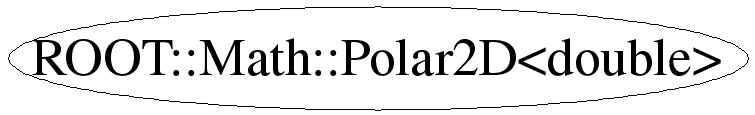# class ROOT::Math::Polar2D<double>

```
Class describing a polar 2D coordinate system based on r and phi
Phi is restricted to be in the range [-PI,PI)

@ingroup GenVector

```

## Function Members (Methods)

public:
 ~Polar2D() void GetCoordinates(ROOT::Math::Polar2D::Scalar& r, ROOT::Math::Polar2D::Scalar& phi) const ROOT::Math::Polar2D::Scalar Mag2() const void Negate() bool operator!=(const ROOT::Math::Polar2D& rhs) const ROOT::Math::Polar2D& operator=(const ROOT::Math::Polar2D& v) bool operator==(const ROOT::Math::Polar2D& rhs) const ROOT::Math::Polar2D::Scalar Phi() const ROOT::Math::Polar2D Polar2D() ROOT::Math::Polar2D Polar2D(const ROOT::Math::Polar2D& v) ROOT::Math::Polar2D Polar2D(double r, double phi) ROOT::Math::Polar2D::Scalar R() const void Rotate(double angle) void Scale(double a) void SetCoordinates(ROOT::Math::Polar2D::Scalar r, ROOT::Math::Polar2D::Scalar phi) void SetPhi(const double& phi) void SetR(const double& r) void SetX(ROOT::Math::Polar2D::Scalar x) void SetXY(ROOT::Math::Polar2D::Scalar x, ROOT::Math::Polar2D::Scalar y) void SetY(ROOT::Math::Polar2D::Scalar y) ROOT::Math::Polar2D::Scalar X() const double x() const ROOT::Math::Polar2D::Scalar Y() const double y() const
private:
 static double pi() void Restrict()

## Data Members

private:
 double fPhi double fR

## Class Charts## Function documentation

Polar2D & operator=(const ROOT::Math::Polar2D<double>& v)
```assignment operator

```
void SetCoordinates(ROOT::Math::Polar2D<double>::Scalar r, ROOT::Math::Polar2D<double>::Scalar phi)
```Set internal data based on 2 Scalar numbers

```
`{ fR=r; fPhi=phi; Restrict(); }`
void GetCoordinates(ROOT::Math::Polar2D<double>::Scalar& r, ROOT::Math::Polar2D<double>::Scalar& phi) const
```get internal data into 2 Scalar numbers

```
`{r=fR; phi=fPhi;}`
Scalar R()
`{ return fR;}`
Scalar Phi()
`{ return fPhi; }`
Scalar X()
`{ return fR*std::cos(fPhi);}`
Scalar Y()
`{ return fR*std::sin(fPhi);}`
Scalar Mag2()
`{ return fR*fR;}`
void SetR(const double& r)
``` setters (only for data members)

set the r coordinate value keeping phi constant

```
void SetPhi(const double& phi)
```set the phi coordinate value keeping r constant

```
Restrict()
void SetXY(ROOT::Math::Polar2D<double>::Scalar x, ROOT::Math::Polar2D<double>::Scalar y)
``` all values using cartesian coordina

```
double pi()
`{ return M_PI; }`
void Scale(double a)
```cale by a scalar quantity - for polar coordinates r change

```
Negate()
void Rotate(double angle)
```ate the vec

```
bool operator==(const ROOT::Math::Polar2D<double>& rhs) const
```Exact equality

```
bool operator!=(const ROOT::Math::Polar2D<double>& rhs) const
`{return !(operator==(rhs));}`
T x()
``` ============= Compatibility section ==================
The following make this coordinate system look enough like a CLHEP
vector that an assignment member template can work with either
```
`{ return X();}`
T y()
`{ return Y();}`
void SetX(ROOT::Math::Polar2D<double>::Scalar x)
``` ============= Specializations for improved speed ==================
(none)
====== Set member functions for coordinates in other systems =======
```
void SetY(ROOT::Math::Polar2D<double>::Scalar y)

Last update: root/mathcore:\$Id: Polar2D.h 21503 2007-12-19 17:34:54Z moneta \$
Copyright (c) 2005 , LCG ROOT MathLib Team and *

This page has been automatically generated. If you have any comments or suggestions about the page layout send a mail to ROOT support, or contact the developers with any questions or problems regarding ROOT.